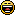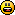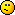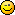# Math quiz that's not really a math quiz--LOL

1. Okay, there is a trick to this one; you must think outside the box.  (Remember, I'm not a mathematician, and I suck at math...so this is more of a creative thinking than a math quiz.)

What is the sum of the 1st G, squared?

1. How could something squared or a prime be a prime? What is this 1st G thing?

1. Not knowing what 1st G is, I named G as 7 (7th letter in the alphabet). 7 times 7 is 49. The sum of those digits is 13.

1. Ooh, I like this. I'd say 140 then.
1^2 + 2^2 + ... + 7^2

2.0
Bob Bambergposted 5 years ago

64

1. Nope--sorry.  Hint:  you have to think in terms of games...3. Pretty deep into Mathematics here, what is a 1st G?

4. 6760000....maybe? Am I on the right track?

5. I don't think the answer is a number. It's not maths. It's a game.

Even the question doesn't make sense if you substitute 1st G with an actual number. What is the sum of 4, squared? You'd need to say 'what is the sum of 2+2, squared?'... Wouldn't you?

So it's something else.

1. I'm with you.  Perhaps the answer is "checkmate".

2. I was thinking it's something on the lines of the sum of the 1st grand squared. Like 1 squared + 2 squared plus ... up to a grand. Makes sense that it's not Maths though.

6. Okay; perhaps this was a bit too obsure.

BIG hint:  the starting point is "the first (number) under G"  ....  again, think of games using that letter...

1. There is no sum to the 1st G squared. The letter G in Scrabble has a value of 2 points. Two squared (2 x 2) is 4. Four is a single digit. If the answer had two or more digits, they could be added together to come up with the sum. Therefore, there is no sum to the 1st G squared.

2. What is the sum of the first G squared? If G is 2, this translates to: What is the sum of the first 2 squared. 1^2 + 2^2? So the answer is 5?

1. No--incorrect assumption.  the first G is not 2.  (and even it it were, I get 4--because, at least when I went to school, a number squared meant simply a number times itself, so 2*2=4.) However, the number we are looking for is not 2
Hint #2:  it's a 2-digit number.

1. I hope it is a cards game in which the A or Ace card represents 11. So, the square of it is 121 and the sum of it is 4.

2. The sum of the first N squared is what I did though. Like here: http://mathforum.org/library/drmath/view/56920.html

What you seem to be asking for is the sum of the digits of G squared and not the sum of the first G squared? Maybe Venkat has it right.

7. Does it have anything to do with gin rummy? I'm stumped with "first G" which implies there's more than one.8. Okay...I guess I really want too far out of the box with this.  Final clue, and this pretty much gives away the starting number.  Think of a game very popular with senior citizens, and which is often hosted by non-profit groups as a fundraiser...

9. Okay--I'll give up the gig.

Think of B I N G O (a game popular with senior citizens) ...  the first (number under) G is the number you are looking for...that number squared, then, is______

10. Oh. My mother plays bingo. So are you talking about the card? I've Googled images and the numbers are all different. Unless your cards are otherwise in the US.

'1st G' though? There's only one.

1. I don't think they play 90 ball bingo.
Looking online, G is nos 46 to 60. So, 1st G squared would be 46 x 46 = 2,116

1. And 2+1+1+6 = 10. To break it down ever farther, 1+0 = 1.

1. You started out correctly, Shauna, but went off track by breaking it down.  ;-)

2. And..YOU got a BINGO!  Congrats to Marketing Merit!

It never was (really) about the math question; it was about thinking outside the box enough to identify the game from which the starting number was chosen.

1. So, Marketing merit's answer is correct and the sum of it is 10.

The first G is 46. Its square is 2116. And, the sum of it is 10.

11. 16or 64, yes, 64

12. @  Venkatachari M:
By "sum," I only meant the answer to the number squared.  I was unaware that using the word " sum" meant any further calculation to  anyone else.
I did preface the whole thing by stating, "I'm no mathematician."

1. The word "Sum" means a total. A total is the addition of some numbers. If you meant the answer to be only the square of a particular number, then, you need to say simply the square of it and not the sum of the square. So, your question created some ambiguity. But, don't mind it.

1. There does appear to be some difference of opinion as regards what the term sum of means.

One of the subjects I tutor to adults in the UK, is numeracy. So, if I asked, "what the sum of 6 + 5" I would expect an answer of 11 and not 2.

Similarly, if I asked for "the sum of 5 squared + 2" I would expect an answer of 27 and not 9.

If I wanted a single digit, I would ask the learners to keep adding the integers, until they reached that point. This is referred to as the digit sum.

1. Yes, that is what I also said.

13. Oh well, being in the UK, I stood no chance at all at getting it rightThere is no connection between the word BINGO (if it is actually printed on the card at all) and the numbers beneath.

https://en.wikipedia.org/wiki/Bingo_(United_Kingdom)

Incidentally, my mother has won £1200 (\$1800 - ish) at Bingo in the last five weeks1. In the US, the word "BINGO" is printed at the top of the cards, so there is a connection.1. Oops! Didn't realize there was a winner. Well, I must tell you that I found a BINGO card where the number under G was 56 so I squared it and got the # above. I was on the right track it seems.1. LOL, Janshares; the trick was the FIRST number that comes under G, and the numbers under G run from 46 through 60, inclusive.   ;-)
Thanks for playing.

1. It was tricky, but fun.working